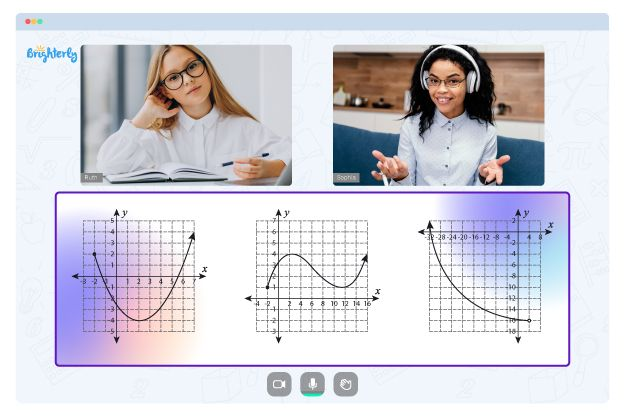# Points Lines and Planes Worksheets

Geometric constructions, proofs, and problem-solving approaches frequently use planes, points, and lines. The basic geometric concepts of points, lines, and planes are the foundation for numerous geometric ideas and principles. Understanding the properties and behavior of geometric shapes and figures also requires the knowledge of these concepts.

## A Point

A point is an element in space without size or shape that is denoted by a dot. Lines and planes, among other geometrical figures, are defined by points. For instance, if we have two points, A and B, we can connect them in a straight path to create a line.

## A Line

A line is a straight path that can go in either direction indefinitely. It is typically identified by two points on a line and is symbolized by an arrow. The two points are referred to as the line’s endpoints.

## A Plane

A plane is a flat surface that can be extended in any direction. It is typically denoted by three non-collinear points and is represented by a rectangle. The three points are referred to as the plane’s vertices.

## Worksheets

Points lines and planes worksheet answers PDF is a resource for students to practice and reinforce the knowledge on lines and points. The exercises and problems in these worksheets typically cover a variety of point, line, and plane topics. The tasks on identifying points, lines, and planes may be included in some worksheets, while others focus on teaching students to construct geometric figures or solving geometric problems.

Math for Kids

Is Your Child Struggling With Math?
1:1 Online Math TutoringPoints lines and planes worksheets answers help students practice and reinforce these ideas, becoming vital resources in their journey to understand geometry better. With the help of the points lines planes worksheets, students can learn topics in a well-organized way and retain the information they’ve learned. Students can also have fun studying math thanks to these points lines and planes worksheet answers, which help them work at their own pace, following a flexible schedule to solve the questions.### Points Lines and Planes Worksheets PDF

Points Lines And Planes Worksheet Answers Pdf### Points Lines and Planes Worksheets PDF

Points Lines Planes Worksheet### Points Lines and Planes Worksheets PDF

Points Lines And Planes Worksheets Answers### Points Lines and Planes Worksheets PDF

Points Lines And Planes Worksheet

Need help with Function Graph?• Does your child struggle with mastering of function graph?
• Try studying with an online tutor.

Is your child having difficulties with understanding function graph? An online tutor could provide the necessary guidance.